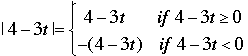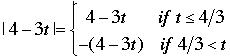SEARCH HOMEMath Central Quandaries & QueriesQuestion from ryan, a student: Good day! We we're given this assignment in algebra concerning linear inequalities with absolute value. In this number I'm confused how to isolate the absolute value, considering there is a variable in the absolute value and there is a variable in the outside. 2+5 |4-3t|+t ≤ -3-4tHi Ryan,

|4 - 3t| is either 4 - 3t or it's -(4 - 3t) and I would use this to split the problem into two inequalities right from the start. To be more precise.

4 - 3t ≥ 0 is equivalent to t ≤ 4/3 and 4 - 3t < 0 is equivalent to 4/3 < t and hence.

So now separate the problem into two situations.

Part 1: t ≤ 4/3

In this case |4 - 3t| = 4 - 3t so the inequality becomes

2 + 5 (4-3t)+ t ≤ -3 -4t

Solve for t. To be a solution of the original problem t must satisfy both the inequality you just obtained and the inequality t ≤ 4/3.

Part 2: t < 4/3

In this case you have |4 - 3t| = -(4 - 3t) so substitute this into the original equation and proceed as in part 1.

Write back if you have any difficulties in completing the solution.

PennyMath Central is supported by the University of Regina and The Pacific Institute for the Mathematical Sciences.